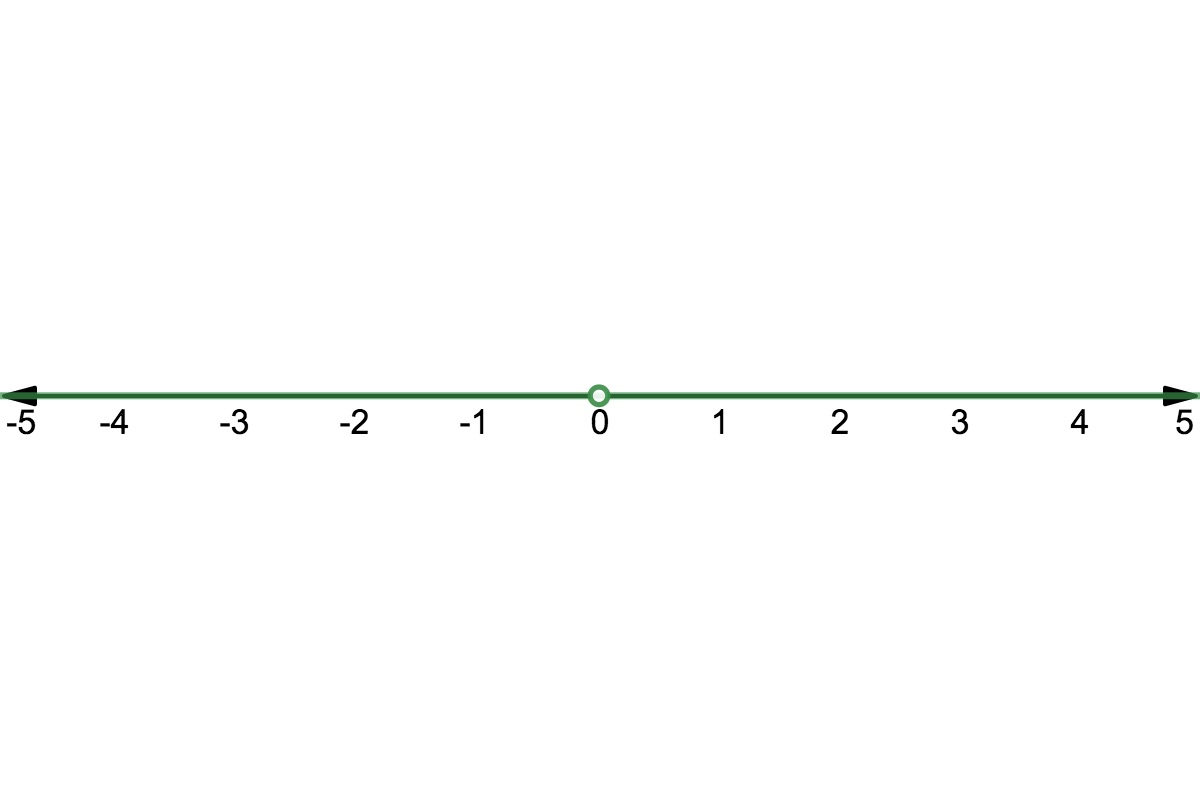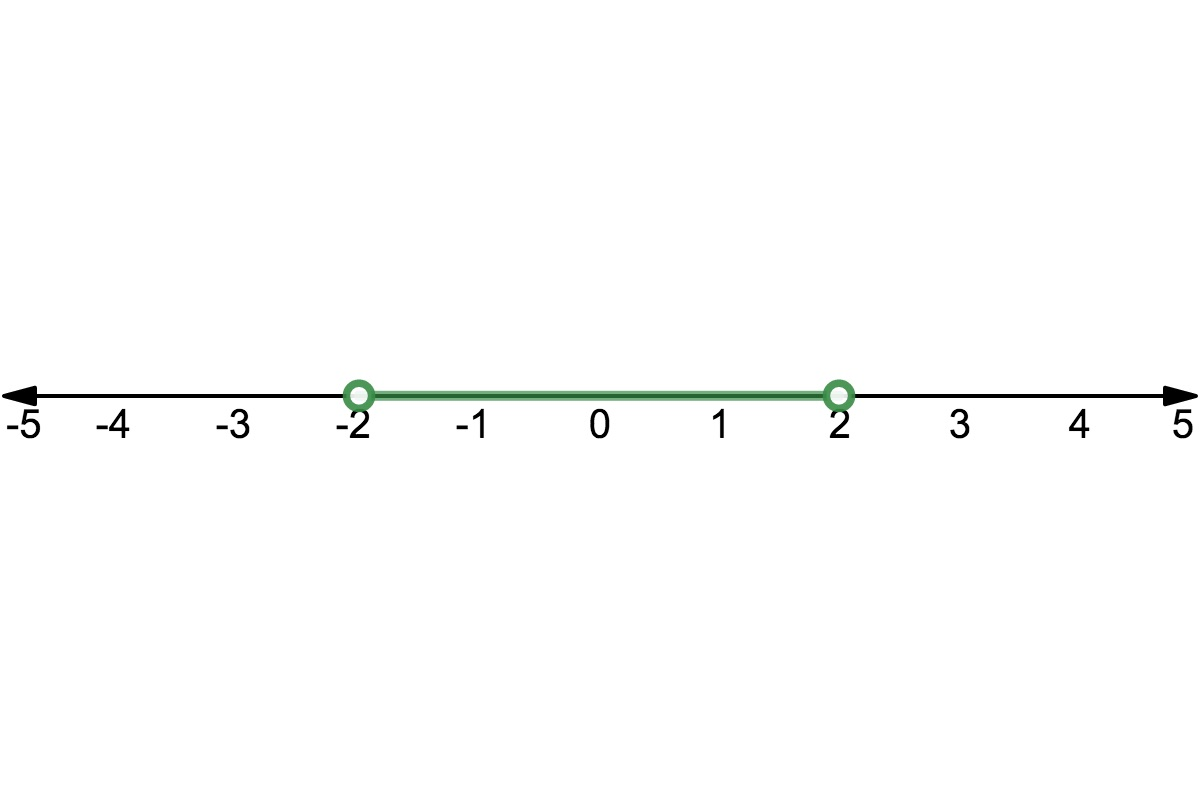# LINR 2 | Lesson 2 | Try This! (Absolute Value Inequalities) Solutions

1. $$| x | ≥ 3$$  means all numbers  3 or more away from 0.

$$x ≥ 3$$ or $$x ≤-3$$

1. All values of $$x$$ excluding 0.1. $$-2 < x < 2$$
1.There are no values of $$x$$ for which the absolute value is a negative number.

For more on Absolute Value go to Desmos.com